Courses
Courses for Kids
Free study material
Free LIVE classes
More

# Parallel First Order Reaction Kinetics for JEELIVE
Join Vedantu’s FREE Mastercalss

## Kinetics of Reaction

Chemical kinetics, usually referred to as reaction kinetics, is a branch of physical chemistry that deals with the speeds of chemical reactions. It contrasts with thermodynamics, which deals with the direction in which a process occurs but does not reveal the rate of the process. Chemical kinetics is the study of how experimental conditions affect a chemical reaction's rate and reveal details about its mechanism and transition stages. It also involves the development of mathematical models that may be used to represent the properties of a chemical reaction.

## Complex Reactions and Its Types

The primary chemical process is occasionally accompanied by a few side reactions. Since these reactions do not occur in a single step, they are referred to as complex reactions. In other words, rather than occurring in a single step, such complicated reactions happen in a sequence of steps, and the overall reaction rate follows the reaction's stoichiometric equations. The following types of complex reactions occur:

• Consecutive reactions

• Parallel reactions

• Reversible or opposing reactions

## What are Parallel Reactions?

In these reactions, the reactant takes two or more different pathways, producing one or more products.

By adjusting the circumstances, such as pressure, temperature, or catalyst, the preferential rate of such can be altered. The major or primary reaction is the one in which the greatest yield of the products is achieved.

The other reactions (or reactions) are referred to as side or parallel reactions.

For example,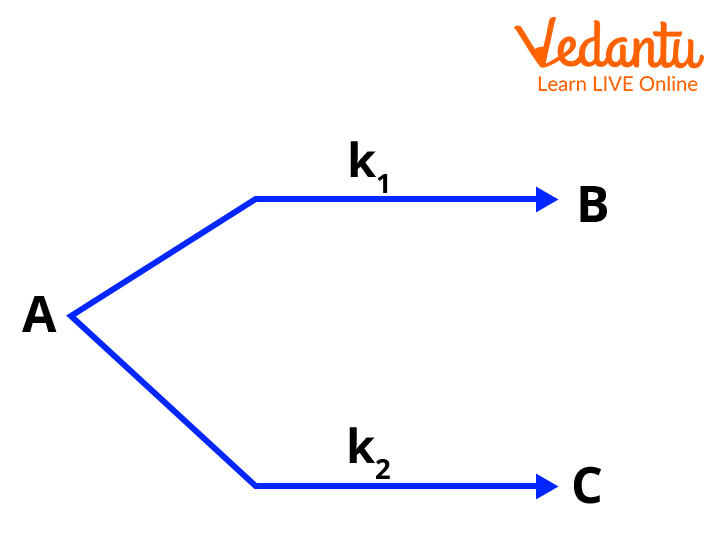Representation of parallel reaction

In the given example of parallel reaction,  A converts to B and C at the same time.

## Kinetics of Parallel Reaction

In the above mentioned process, reactant A yields the products B and C separately in two distinct reactions with the corresponding rate constants k1 and k2. A$\rightarrow$ B will be the principal reaction and A $\rightarrow$C will be the side or parallel reaction if k1 > k2. Suppose both of these reactions are of first order at time t, and A's concentration is $[A]$ at time t, the expressions for the differential rates will be,

\begin{align} &\mathrm{r}_{1}=\dfrac{-\mathrm{d}[\mathrm{A}]}{\mathrm{dt}}=\mathrm{k}_{1}[\mathrm{~A}] \\ &\mathrm{r}_{2}=\dfrac{-\mathrm{d}[\mathrm{A}]}{\mathrm{dt}}=\mathrm{k}_{2}[\mathrm{~A}] \end{align}

The overall rate of disappearance of A is as follows

$\dfrac{-\mathrm{d}[A]}{\mathrm{d} t}=r_{1}+r_{2}=(\mathrm{k}_1+\mathrm{k}_2)[\mathrm{A}]=\mathrm{k}^{\prime}[\mathrm{A}]$

Integrating equations, we get

\begin{align} &\ln \dfrac{\left[\mathrm{A}_{\circ}\right]}{[\mathrm{A}]_{\mathrm{t}}}=\mathrm{k}^{\prime} \mathrm{t}=(\mathrm{k}_1+\mathrm{k}_2) \mathrm{t} \\ &\left[\mathrm{A}\right]_t=\left[\mathrm{A}\right]_{0} \mathrm{e}^{-(\mathrm{k}_1+\mathrm{k}_2) \mathrm{t}} \end{align}

where $[A]_0$represents the reactant's initial concentration and $[A]_t$ represents its concentration at time t.

Concentration of B at time t

$\dfrac{\mathrm{d}[\mathrm{B}]}{\mathrm{dt}}=\mathrm{k}_{1}[\mathrm{~A}]=k_{1}[A]_{0} e^{-\left(k_{1}+k_{2}\right)t}$

Integrating B with respect to t

$[\mathrm{B}]=-\dfrac{\mathrm{k}_{1}[\mathrm{~A}]_{0}}{\mathrm{k}_{1}+\mathrm{k}_{2}}\left(\mathrm{e}^{-\left(\mathrm{k}_{1}+\mathrm{k}_{2}\right) \mathrm{t}}\right)+\mathrm{c}_{1}$

At t=0 B=0

$\mathrm{c}_{1}=\dfrac{\mathrm{k}_{1}[\mathrm{~A}]_{0}}{\mathrm{k}_{1}+\mathrm{k}_{2}}$

We get

$[\mathrm{B}]=\dfrac{\mathrm{k}_{1}[\mathrm{~A}]_{0}}{\mathrm{k}_{1}+\mathrm{k}_{2}}\left(1-\mathrm{e}^{-\left(\mathrm{k}_{1}+\mathrm{k}_{2}\right) \mathrm{t}}\right)$

Similarly, solving this we get

$[\mathrm{C}]=\dfrac{\mathrm{k}_2 [ \mathrm{A}]_{0}}{\mathrm{k}_1+\mathrm{k}_2}\left(1-\mathrm{e}^{-(\mathrm{k}_1+\mathrm{k}_2) \mathrm{t}}\right)$

Concentration of B and C at time t is given by  the formula

\begin{align} &{[\mathrm{B}]=\dfrac{\mathrm{k}_1[\mathrm{~A}]_{0}}{\mathrm{k}_1+\mathrm{k}_2}\left(1-\mathrm{e}^{-(\mathrm{k}_1+\mathrm{k}_2) \mathrm{t}}\right)} \\ &{[\mathrm{C}]=\dfrac{\mathrm{k}_2[\mathrm{~A}]_{0}}{\mathrm{k}_1+\mathrm{k}_2}\left(1-\mathrm{e}^{-(\mathrm{k}_1+\mathrm{k}_2) \mathrm{t}}\right)} \end{align}

The ratio of concentration of  B to concentration of C is given by

$\dfrac{[\mathrm{B}]}{[C]}=\dfrac{k_1}{k_2}$

## Graph of Parallel Reaction

As the reaction proceeds, the concentration of A decreases and concentration of B and C increases as k1>k2 the concentration of B increases more rapidly than concentration of C.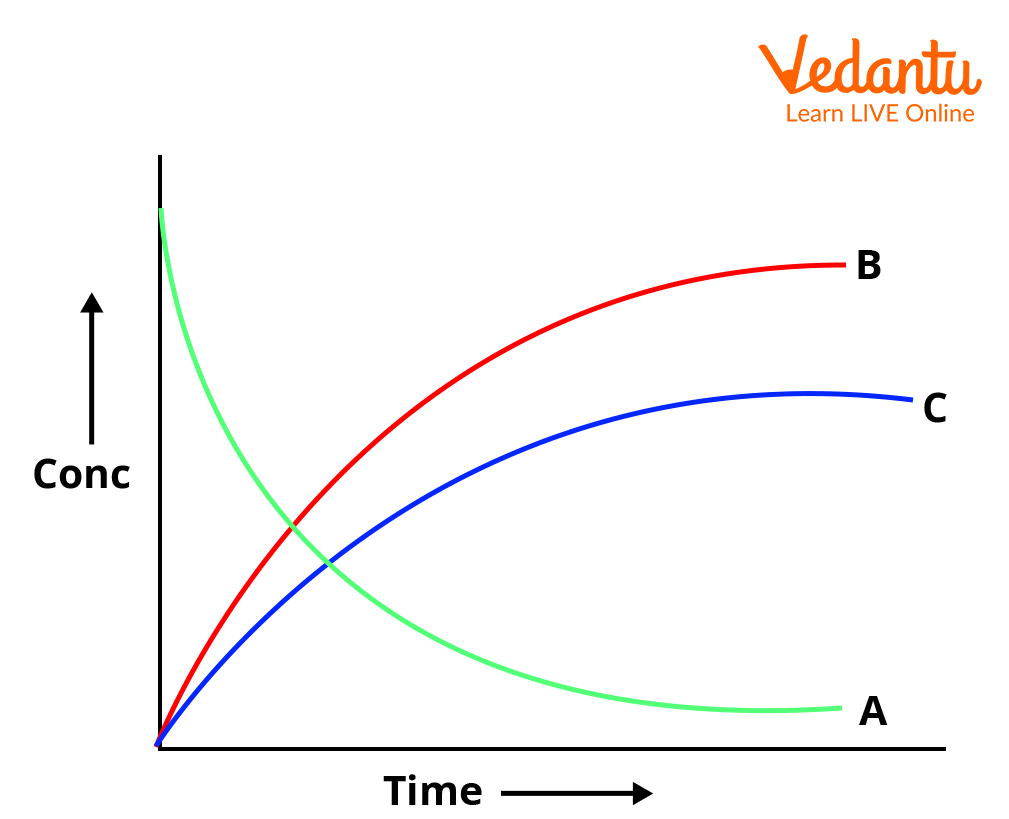Graph of parallel reaction

## Examples of Parallel Reactions

Some parallel reaction examples are

•  Reaction of ethyl bromide with potassium hydroxide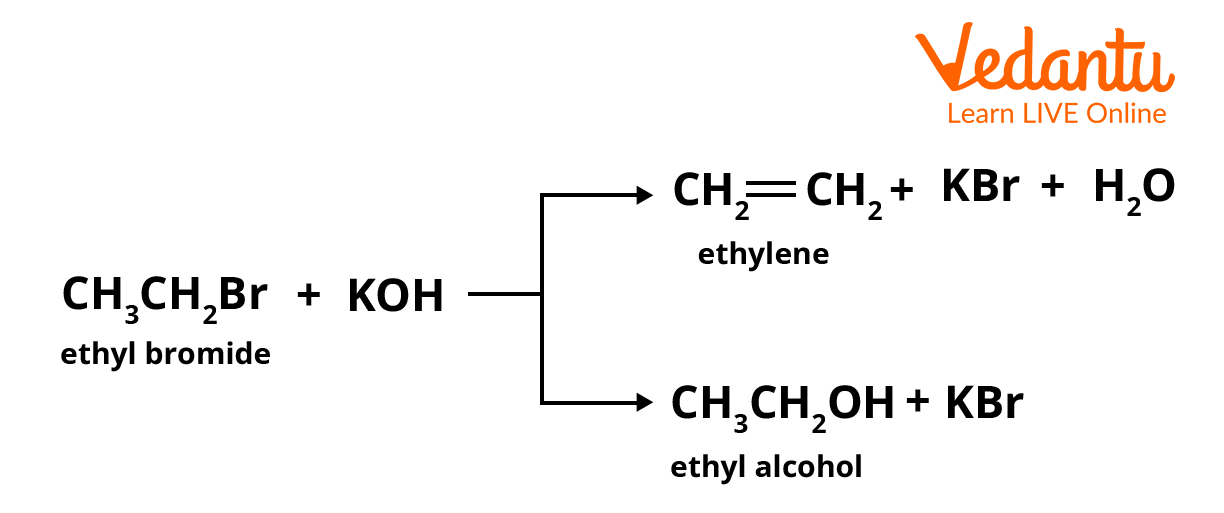Parallel reaction of ethyl bromide

•  Dehydration of 2-methyl–2–butanol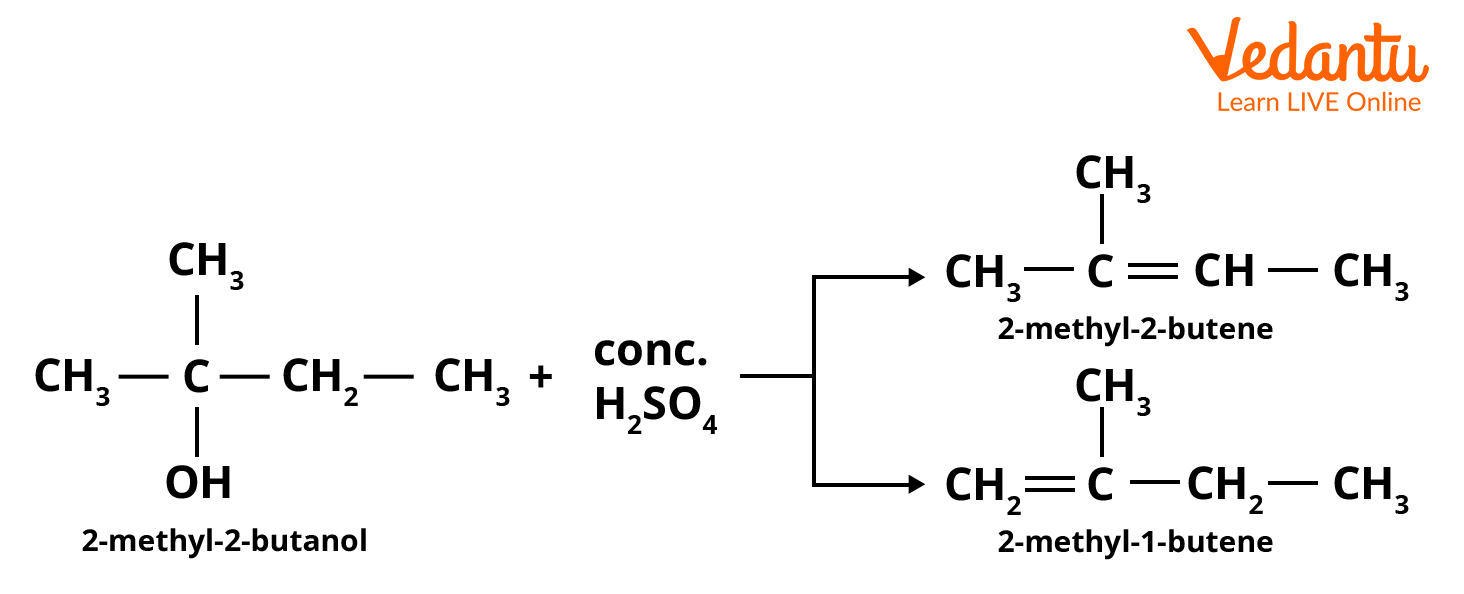Parallel reaction 2-methyl-2-butanol

## Consecutive Reactions

Consecutive reactions are those in which the final product is created through one or more intermediate steps. Sequential reactions is another name for these. In these reactions, the outcome of one elementary reaction serves as the reactant for another elementary reaction.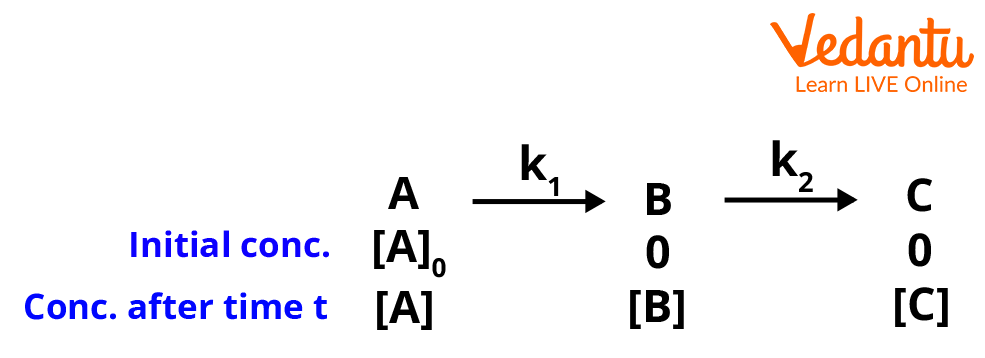Representation of Consecutive reaction

In the above reaction, reactant A and intermediate B combine to generate the product C. The rate constants for this reaction are k1 for the first stage and k2 for the second stage.

The size of these two rate constants determines the net or overall rate of reaction. For each species in the given reaction, the initial concentration and the concentration after time t are shown. The initial concentration is given as :

$[A]_0 = [A] + [B] + [C]$

## Graph For Consecutive Reaction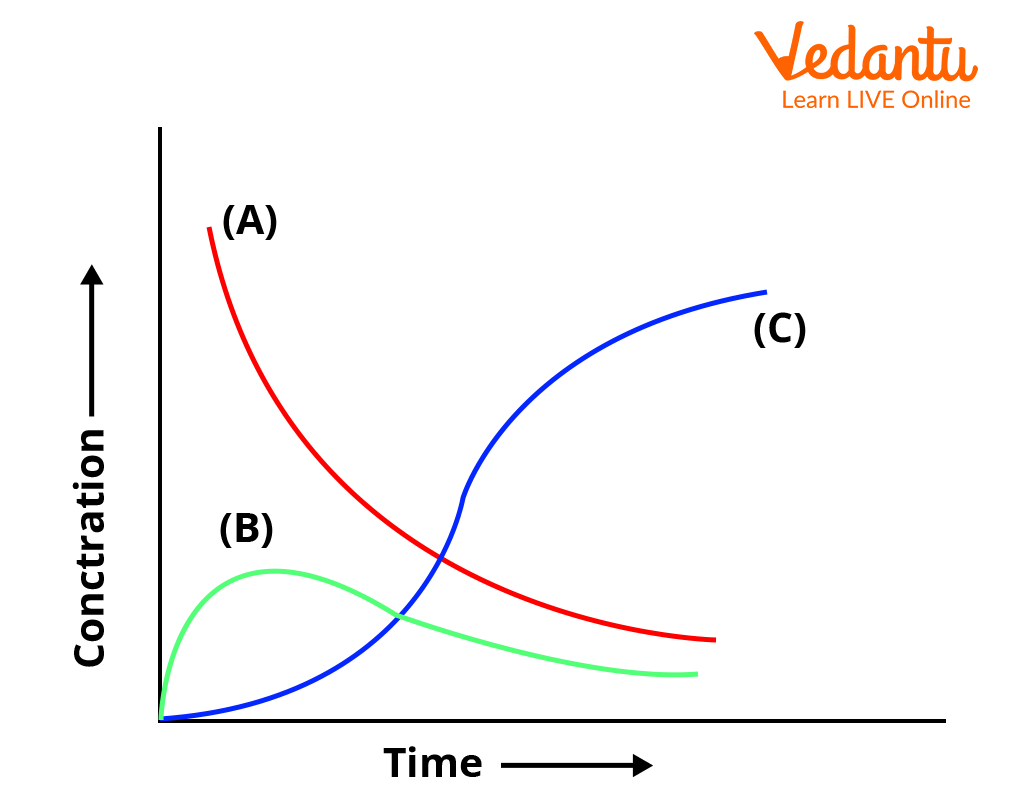Graph for consecutive reaction

The concentration of A decreases exponentially; the concentration of B first rises and then falls; and the concentration of C rises over time (from zero). It eventually reaches a value equal to $[A]_0$ (the initial concentration A), when all A has changed into the final product C.

## Examples for Consecutive or Sequential Reactions

• Decomposition of dimethyl ether is gaseous phase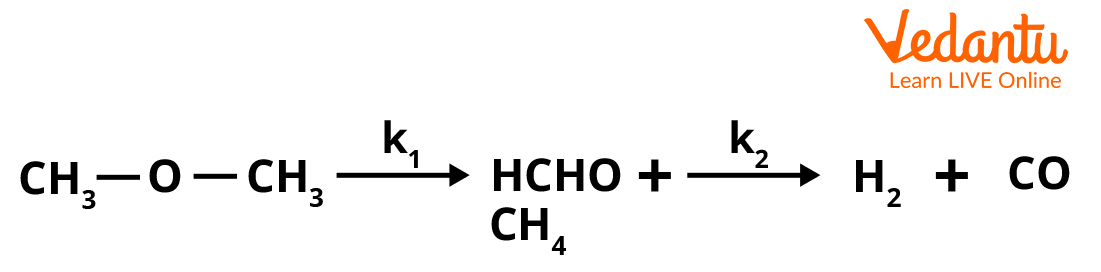Consecutive reaction of dimethyl ether

• Decomposition of Ethylene oxide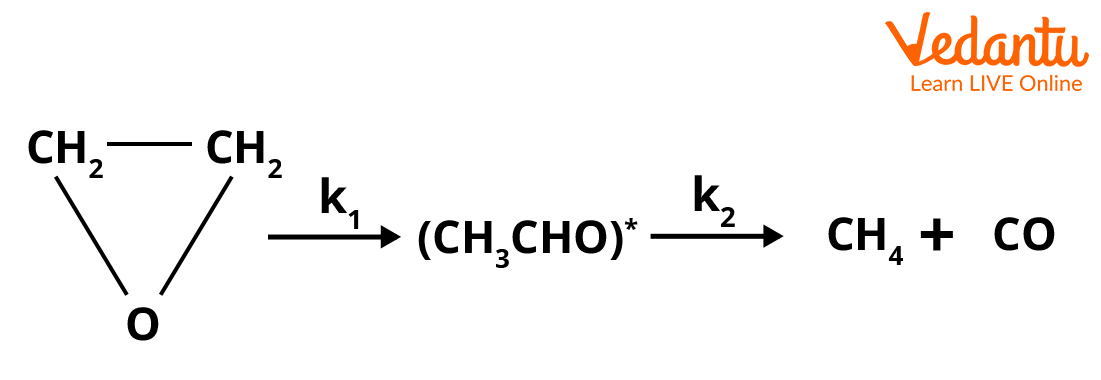Consecutive reaction of ethylene oxide

The above two reactions are examples of consecutive reactions.

## Conclusion

Chemical kinetics, usually referred to as reaction kinetics, is a discipline of physical chemistry concerned with the speeds of chemical reactions. The primary chemical process is occasionally accompanied by a few side reactions. Since these reactions do not occur in a single step, they are referred to as complex reactions. Complex reactions consist of consecutive reactions, parallel reactions, reversible or opposing reactions. In parallel reactions, the reactant takes two or more different pathways, producing one or more products.

By adjusting the circumstances, such as pressure, temperature, or catalyst, the preferential rate of such can be altered. The major or primary reaction is the one in which the greatest yield of the products is achieved. The other reaction (or reactions) is referred to as side or parallel reaction.

Last updated date: 26th Sep 2023
Total views: 3k
Views today: 0.03k

## FAQs on Parallel First Order Reaction Kinetics for JEE

1. What is a pseudo first order reaction?

A pseudo first order reaction can be defined as a second-order or bimolecular reaction that is made to behave like a first-order reaction. This reaction occurs when one reacting material is present in great excess or is maintained at a constant concentration compared with the other substance.

For example, hydrolysis of cane sugar

$\mathrm{C}_{12} \mathrm{H}_{22} \mathrm{O}_{11}+\mathrm{H}_{2} \mathrm{O} \rightarrow \mathrm{C}_{6} \mathrm{H}_{12} \mathrm{O}_{6}+\mathrm{C}_{6} \mathrm{H}_{12} \mathrm{O}_{6}$

We can see that water has a very high concentration, and so does not vary much during the reaction. As a result, the rate of the reaction is independent of the change in H2O concentration. The rate equation can be given as,

$\text { Rate }=\mathrm{k}\left[\mathrm{C}_{12} \mathrm{H}_{22} \mathrm{O}_{11}\right]$

2. What are opposing reactions?

In opposing or reversible reactions, the products that are produced also react back to form the reactants. Initially, the rate of forward reaction is quite high. However, it gradually declines over time, while the rate of the backward or reverse reaction is zero and gets larger as the reaction progresses. When this stage is reached, two rates are equalized and a chemical equilibrium is established. Its nature is dynamic as all the species are reaching at the rate at which they are being formed.